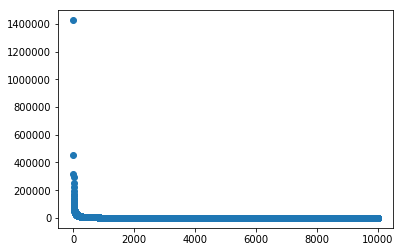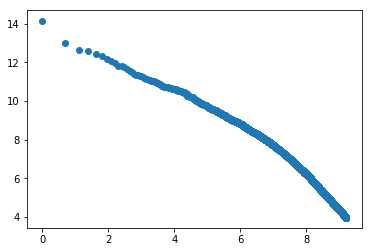In :
import numpy
import pandas
from matplotlib import pyplot


### All of statistics in one sentence¶

Some of the variability in a dataset is predictable, some is not.

[Notation review: $Y$ is a random variable. $\bar{Y}$ ("y bar") is the mean of $Y$. $\hat{Y}_i$ ("y hat") is an estimate of $Y_i$ based on a specific dataset.]

A linear model is one of the simplest ways of describing relationships between variables. We have one or more input predictors $X$ and an output $Y$. The linear model says that a good guess for $Y_i$ is $\hat{Y}_i = mX_i + b$. The gap between the real $Y_i$ and the guess $\hat{Y}_i$ is the error $\epsilon_i$ or the "residual".

The fact that a model doesn't perfectly predict the output variable doesn't mean that that extra gap isn't predictable, it just means that it's not predictable as a linear function of the given input variables.

It is important to remember that for a linear model what we care about is the vertical distance from the prediction line to the point, not the shortest line perpendicular to the prediction line.

### Derivation of the least squares rule¶

Assume we have a set of pairs $X_i, Y_i$, and that these values are centered, so that the mean $X_i$ and the mean $Y_i$ are zero.

We want to find a value $m$ such that $mX_i$ is a good predictor of $Y_i$. How can we decide what the best value is?

We can define a "loss" function that tells us how bad a given $m$ is. There are many such functions! The one that is most mathematically convenient is squared loss: $\mathcal{L} = \sum_i (Y_i - mX_i)^2$.

Why is this convenient? To find the optimal value of $m$ we can find the derivative of the loss function with respect to $m$ and set that to zero, then solve for $m$. If the function is a sum of squared terms, the calculus is easy. Absolute values would be harder. (Another connection, which I didn't mention in class, is that $-\mathcal{L}$ is the terms of the log of a product of Gaussians with mean $mX_i$ and variance $\sigma^2 = 1$.)

\begin{align} \frac{d}{dm}\mathcal{L} & = \sum_i 2(Y_i - mX_i)X_i \\ & = 2 \sum_i X_i Y_i - 2 \sum_i mX_i^2 \end{align}

The 2 comes from the derivative of the square. The extra $X_i$ at the end comes from the fact that $(Y_i - mX_i)$ is a function of $m$, so we need to apply the chain rule: the derivative of that is $X_i$ because the $Y_i$ drops out and the $m$ goes away. The second line splits the sum into two terms and multiplies both of them by $2X_i$.

Setting this equal to zero and solving for $m$ gives us

\begin{align} 0 & = 2 \sum_i X_i Y_i - 2 \sum_i mX_i^2 \\ m\sum_i X_i^2 & = \sum_i X_i Y_i \\ m &= \frac{\sum_i X_i Y_i}{\sum_i X_i^2 } \quad \quad !!! \end{align}

where the $m$ can be pulled out of the sum over $i$ because it is the same for each term of the sum, and the 2 drops out because it is on both sides. We are excited to see this result! It is almost exactly the formula we have been using for the estimated slope $\hat{m}$, the "least squares" estimator. The difference is that previously we were explicitly centering the dataset by subtracting the mean from each value:

\begin{align} \hat{m} &= \frac{\sum_i (X_i - \bar{X})(Y_i - \bar{Y})}{\sum_i (X_i - \bar{X})^2 } \end{align}

### The $r^2$ value for two variables¶

Given a set of residuals (errors in prediction) for a linear model (a slope $m$ and intercept $b$), we can define various ways to turn those numbers into a single score that measures the quality of the model. The "squared error" model that we used above is sensitive to outliers, but is mathematically easy to work with. We'll go with it for now.

[Notation note: Most presentations use lots of acronyms that all look like SS?. I find these totally unintuitive and unhelpful and I don't actually use them, so I'm going to skip them from now on.]

How can we measure whether a given linear model is good or not? As in other cases, we can compare the model we estimated to a boring "null" model. In this case, a boring linear model is one that ignores $X_i$ and predicts the mean $\bar{Y}$ all the time. This looks like a horizontal line in our usual cartesian plot. This is a good baseline: we could obviously do worse by setting the slope to infinity, but could we do better?

The $r^2$ metric measures this intuition:

\begin{align} r^2 = 1 - \frac{\sum_i (Y_i - (mX_i + b))^2}{\sum_i (Y_i - \bar{Y})^2} \end{align}

### Examples of $r^2$¶

The numerator in the formula for $r^2$ is zero when there are no residuals in the fitted line. Each point lies exactly along a line with no additional variability. Everything is perfectly systematic, there is no remaining variance to explain. It doesn't matter what the slope of the line is, as long as it's not 0: then the denominator is also 0, which makes your function blow up.

$r^2$ is smallest when the numerator is equal to the denominator. This would happen if the best fit line has slope zero.

### $r^2$ and $r$¶

What is the relationship between the correlation coefficient $r$ and the coefficient of determination $r^2$? The second is, in fact, the square of the first.

Assume that $X$ and $Y$ both have mean 0, so that $X_i - \bar{X} = X_i$. We know that the best $m$ is $\frac{\sum_i X_i Y_i}{\sum_i X^2}$. Can you show that

\begin{align} 1 - \frac{\sum_i (Y_i - (mX_i))^2}{\sum_i Y_i^2} = \frac{(\sum_i X_i Y_i)^2}{(\sum_i X^2)(\sum_i Y^2)} \end{align}

## Straightening curves¶

The linear model works best when $r^2$ is large, and all the points lie along a line. But a lot of real data does not lie along a line. Can we still apply linear regression if the data is curved?

The answer is often yes: if the curve of the data follows a particular function, we can apply the inverse of that function to "straighten it out".

Here's a classic example, Zipf's law. The relationship between the number of occurences of a word (it's token count) and the rank of that word by frequency has a log linear relationship.

In :
word_ranks = pandas.read_csv("word_ranks.tsv", delimiter="\t")

In :
word_ranks.head()

Out:
word tokens docs token_rank
0 the 1425985 153632 1
1 and 454266 130488 2
2 that 317045 109655 3
3 for 293538 110929 4
4 you 249550 75539 5
In :
word_ranks.describe()

Out:
tokens docs token_rank
count 1.000000e+04 10000.000000 10000.00000
mean 1.723093e+03 1068.778500 5000.50000
std 1.713588e+04 4762.633464 2886.89568
min 5.300000e+01 1.000000 1.00000
25% 9.100000e+01 67.750000 2500.75000
50% 1.940000e+02 146.000000 5000.50000
75% 6.732500e+02 516.000000 7500.25000
max 1.425985e+06 153632.000000 10000.00000
In :
pyplot.scatter(word_ranks.token_rank, word_ranks.tokens)
pyplot.show()In :
pyplot.scatter(numpy.log(word_ranks.token_rank), numpy.log(word_ranks.tokens))
pyplot.show()